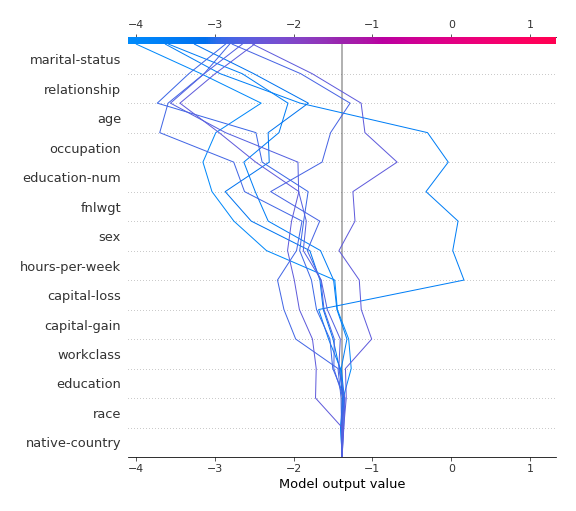# Explainability in AutoML¶

There are three modes of explanations available in `mljar-supervised`. The explanations are controlled by `explain_level` parameter in `AutoML` constructor.

• if `explain_level` is `0` no explanations are produced. Only learning curves are plotted.
• if `explain_level` is `1` the following explanations are produced: learning curves, importance plot (with permutation method), for decision trees produce tree plots, for linear models save coefficients.
• if `explain_level` is `2` the following explanations are produced: the same as `1` plus SHAP explanations.

## Learning curves¶

The learning curves show the evaluation metric values in each iteration of the training. The learning curves are plotted for training and validation datasets. The vertical line is used to show the optimal iteration number, which will be later used for computing predictions. Learning curves are always created.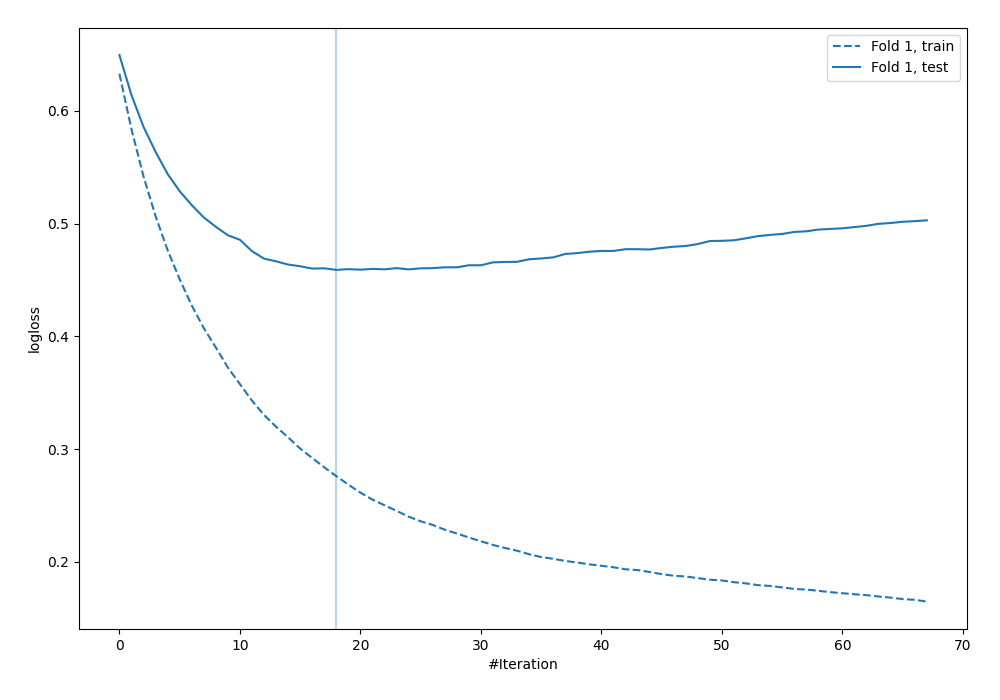## `Decision Tree` Visualization¶

The visualization of the `Decision Tree` is created if `explain_level >= 1`. The `dtreeviz` is used to plot the tree.## `Linear` model coefficients¶

For the `explain_level >= 1` the coefficents of the `Linear` model are saved in the Markdown report. The example of cofficents is presented below.

feature Learner_1
capital-gain 2.28219
education-num 0.843846
age 0.468591
sex 0.468299
hours-per-week 0.369091
capital-loss 0.279562
race 0.104163
education 0.0546121
fnlwgt 0.0545988
native-country 0.0173909
occupation -0.00958272
workclass -0.102386
relationship -0.154081
marital-status -0.358737
intercept -1.51172

## Features Importance¶

The features importance is computed with permutation-based method (using scikit-learn `permutation_importance`). The features importance can be computed to any algorithm (except of course `Baseline`, which doesnt use import features at all). The importance is presented in the plot (top-25 importance features) and saved to the file `learner_*_importance.csv` for all features. It needs `explain_level >= 1`.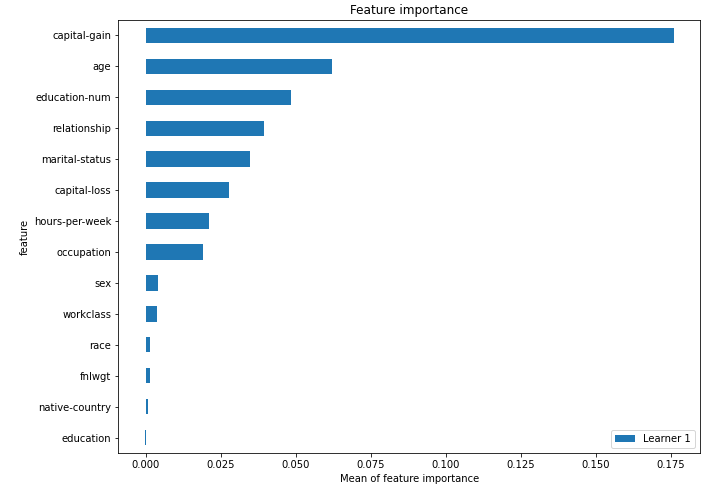## SHAP plots¶

The SHAP explanations are computed if `explain_level = 2`. To compute SHAP explanations the `shap` package is used.

The SHAP explanations are not available for `Baseline`, `Neural Network`, `CatBoost`.

### SHAP importance¶

The SHAP importance example: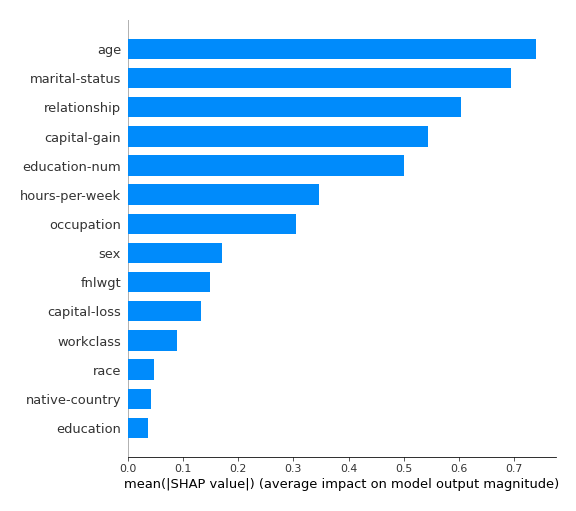### SHAP dependence plots¶

The SHAP dependence plots example: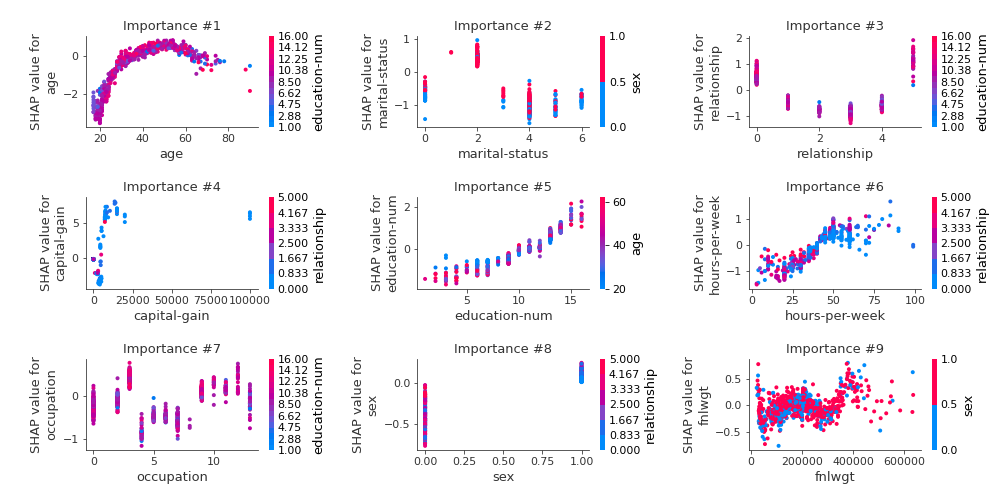### SHAP decision plots¶

For SHAP decisions plots there are created the top-10 worst and best predictions.

The SHAP decision plots example for the best predictions:The SHAP decision plots example for the worst predictions: